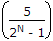# Electronics and Communication Engineering - Digital Electronics - Discussion

Discussion Forum : Digital Electronics - Section 1 (Q.No. 1)
1.
The resolution of an n bit DAC with a maximum input of 5 V is 5 mV. The value of n is
8
9
10
11
Explanation:1000 = 5 or N = 10.

Discussion:
31 comments Page 1 of 4.

Rakshatha priya P said:   5 months ago
Resolution = V/2^N-1.
5mV = 5/2^N-1
2^N-1 = 5/5*10^-3V
2^N-1 = 1/10^-3V
2^N-1 = 10^3V
2^N-1 = 1000
2^N = 1000+1
2^N = 1001.

The nearest power to this value for 2^N is 2^10.
i.e,2^9=512, 2^10=1024, then the value 1024 is nearest to 1001,
then 2^N=2^10,
=> N=10.

Biswas joy said:   2 years ago
Here, the Value of n = summation of total voltage.
(2)

Palaniappan said:   3 years ago
Resolution =(vmax-vmin)/(2^N).
((2^N)-1) = (vmax-vmin/Resolution).
(2^N) = (5-0/5m)+1.
(2^N) = 1001.
Nx(log2) = (log (1001)).
N = (log (1001))/(log2).
N = 9.96.
(4)

Kavya said:   3 years ago
Thanks all for explaining the answer.
(1)

Ashok said:   3 years ago
Thank you all for explaining.
(1)

Dhana said:   3 years ago
Clear explanation. Thanks all.
(1)

Pallavi said:   5 years ago
Nice explanations, thanks All.

Thank you for the clear solution @Sneha.
(1)

Karthickraja said:   5 years ago
5v is 5mv which means the 5v hv n number of 5mv so;
5v=n*5mv,
5v=10*5mv.

Aparna said:   5 years ago
Thanks @Sneha.